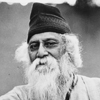FREE ASSISTANCE FOR THE INQUISITIVE PEOPLE
Tutorial Topics
Work Part 2 Energy - Fundamentals
Power - Work, Power and Energy
1289    Arnab De    30/08/2018### POWER

The rate of work by a force is known as power.

The measurement of power is the work done in 1 second by a force.

Let, a force F completes a work w in t seconds. Then the total work done in 1 second is w/t.Thus power spent by a source depends on two factors:

1. The amount of the work done by the source
2. The time taken by the source.

The power of the force cannot measure by the volume of the work, but also how much time taken to finish of that work. As power has no direction. So, it is a scalar quantity.

### Difference between force and power

 Work Power Work done by a force is equal to product of force and displacement of applied point of the force towards the direction of force. Power of source is the rate of doing work by it. Work done does not depend on time. Power is depend of time. S.I. unit of work is joule(J) S.I. unit of power is watt(W)

### Units of power

S.I. Unit: The S.I unit of the power is watt (W).

If 1 joule of work is done in one second, power spent is said to be 1 watt.

Multiple unit of the power are

• Kilowatt (KW)
• Megawatt (MW)
• Gigawatt (GW)

Sub multiple units of power are

• Miliwatt (mW)
• Microwatt (&mu;W)

C.G.S Unit: The C.G.S unit of the power is erg per second (erg s-1).

Relationship between the S.I. unit and C.G.S unit

1 W = 1 J s-1 = 107 erg s-1

F.P.S Unit: The F.P.S unit of the power is Horse Power (HP). It is a unit of power which is widely used in mechanical engineering.

The total power need to move a body of mass 550 pound upward 1 foot in 1 second is measured as 1 horse power.

1HP = 746 watt

1 kW = 1.34 HP

Work Part 2 Energy - Fundamentals
Author DetailsArnab De
I have over 16 years of experience working as an IT professional, ranging from teaching at my own institute to being a computer faculty at different leading institute across Kolkata. I also work as a web developer and designer, having worked for renowned companies and brand. Through tutorialathome, I wish to share my years of knowledge with the readers.
Enter New Comment
Comment History
No Comment Found Yet.We come nearest to the great when we are great in humility.
Rabindranath Tagore
2006
32.39
Today So Far
Total View (Lakh)